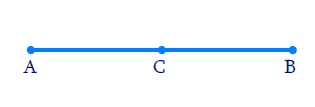In the verge of coronavirus pandemic, we are providing FREE access to our entire Online Curriculum to ensure Learning Doesn't STOP!

# Ex.5.1 Q4 Introduction to Euclids Geometry Solution - NCERT Maths Class 9

Go back to  'Ex.5.1'

## Question

If a point $$C$$ lies between two points $$A$$ and $$B$$ such that $$AC = BC$$, then prove that

\begin{align}AC = \frac{1}{2}AB.\end{align} Explain by drawing the figure.

Video Solution
Introduction To Euclids Geometry
Ex 5.1 | Question 4

## Text Solution

Reasoning:

We are aware that when equals are added to equals, the wholes are equal.

#### Steps:$AC =BC$

Adding $$AC$$ on both sides, we get

\begin{align}&AC+AC=BC+AC \\ &2AC=AB \\\\& \left( \text{BC}\text{+}\text{AC}\,\,\text{coincides with}\text{AB} \right) \\&AC=\frac{1}{2}AB \\ \end{align}

Learn from the best math teachers and top your exams

• Live one on one classroom and doubt clearing
• Practice worksheets in and after class for conceptual clarity
• Personalized curriculum to keep up with school